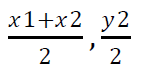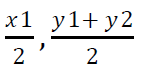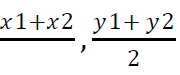# CBSE Class 12 Computer Science Publishing Documents Using Latex MCQs Set B

## MCQ for Class 12 Computer Science Publishing Documents Using Latex

Class 12 Computer Science students should refer to the following multiple-choice questions with answers for Publishing Documents Using Latex in standard 12. These MCQ questions with answers for Grade 12 Computer Science will come in exams and help you to score good marks

### Publishing Documents Using Latex MCQ Questions Class 12 Computer Science with Answers

Question: The 7_______next inside one another and have an integer level assigned to them, with part having a level of 1, chapter a level of 0, section a level of 1 and so on.

a) Lists

b) Details

c) Contents

d) Elements

Question: The parts, chapters, sections are______automatically by LaTeX.

a) Numbered

b) Listed

c) Displayed

d) Edited

Question: The________can also move chapters, sections and subsections without having to bother about remembering.

a) Programmer

b) Author

c) Writer

d) Essayist

Question: The_______are numbered in Roman numerals (I, II, III, and so on).

a) Chapters

b) Sections

c) Parts

d) Subsections

Question: The chapters, sections, subsections, etc. are_______in Arabic numerals (1, 2, 3, and so on).

a) Numbered

b) Listed

c) Displayed

d) Edited

Question: All chapters after the special________command get uppercase numbering (A, B, C, and so on).

a) \parts

b) Mist

c) \appendices

d) \appendix

Question: The_______command is to be used only once.

a) \parts

b) Mist

c) \appendices

d) \appendix

Question: The pages in the_______are numbered using Roman numerals.

a) Front matter

b) Back matter

c) Main matter

d) Whole matter

Question: The pages in the main matter and back matter are numbered in_________numerals with the numbering restarting from 1.

a) Roman

b) Mathematical

c) Decimal

d) Arabic

Question: By default elements are assigned number up to level 2 that is up to_______

a) Chapters

b) Paragraphs

c) Parts

d) Sub subsections

Question: _______and further divisions are not assigned numbers.

a) Chapters

b) Paragraphs

c) Parts

d) Sub subsections

Question: In example, the command "\set counter{secnumdepth}{3}"

a) Ensures that elements up to depth 3 are assigned numbers.

b) Ensures that elements up to level 3 (sub subsection) are assigned numbers

c) Ensures that elements up to counter 3 (sub counter)are assigned numbers.

d) Ensures that elements up to secnumdepth 3 are assigned numbers

Answer: Ensures that elements up to level 3 (sub subsection) are assigned numbers

Question: Elements are assigned a number formed by appending a period (.)

a) Period (.)

b) Colon (:)

c) Semi colon (;)

d) Hash (-)

Question: _________are an exception as their assigned number does not have a part number and period in front of them.

a) Chapters

b) Sections

c) Parts

d) Subsections

Question: ________are assigned simple Arabic numeral numbers.

a) Chapters

b) Sections

c) Parts

d) Subsections

Question: A well-formatted table of contents (TOC) is generated automatically from the elements titles by LaTeX when it encounters the command________

a) \TOC

b) \tableofcontents

c) \tableOC

d) \toContents

Question: By default a_______has entries up to level 2 (subsection).

a) \TOC

b) \tableofcontents

c) \tableOC

d) \toContents

Question: TOC default entries can be changed by altering the value of another built-in counter_________

a) Todepth

b) Tocdepth

c) Counterdepth

d) Setdepth

Question: LaTeX processes the source file..........from the beginning to end in a single pass.

a) Randomly

b) In line

c) Sequentially

d) Cautiously

Question: LaTeX produces the output file________

a) Randomly

b) In line

c) Sequentially

d) Cautiously

Question: In the first run LaTeX collects information about the document structure and stores it in_______files.

a) Extra

b) Complementary

d) Supplementary

Question: In the second run, LaTeX picks up the correct information from the supplementary files in the beginning to produce the correct_________

a) TOC

b) Table of content

c) Table

d) Content

Question: Just like the______LaTeX can also maintain list of figures, list of tables, cross references, bibliography, and glossary or index automatically.

a) TOC

b) Table of content

c) Table

d) Content

Question: In the SciTE editor, the file can be saved using______menu option.

a) File → Build

b) File → Save

c) File → Save as

d) File → Edit

Question: In the SciTE editor, the file can be compiled using_______menu option.

a) Tools → Build

b) File → Save

c) File → Save as

d) Tools → Edit

Question: In the SciTE editor, the shortcut key for compilation is_______

a) F8

b) F4

c) F7

d) F5

Question: In the SciTE editor, the last line "Exit code: 0" is_______in color.

a) Yellow

b) Black

c) Red

d) Blue

Question: In the SciTE editor, the file can be viewed in the default document viewer by selecting_______menu option.

a) Tools → Edit

b) Tools → New

c) Tools → Build

d) Tools → Go

Question: In the SciTE editor, the shortcut key for viewing in the default document is_______

a) F8

b) F4

c) F7

d) F5

Question: In LaTeX documents, a paragraph is typed in a continuous flow without pressing_________key.

a) Tab

b) Enter

c) Shift

d) Delete

Question: LaTeX usually avoids breaking a word in______parts.

a) One

b) Two

c) Three

d) Four

Question: LaTeX has a hyphenation algorithm to decide the most appropriate way of breaking the word with a______

a) Hyphen (-)

b) Tilde(~)

c) Underscore( _ )

d) Colon(:)

Question: LaTeX uses the_______character to denote a non-breaking space.

a) Hyphen .(-)

b) Tilde(~)

c) Underscore( _ )

d) Colon(:)

Question: LaTeX divides font families into______categories.

a) One

b) Two

c) Three

d) Four

Question: ________(also called serif) font have a tiny line or curve called serif at the end of the strokes lines.

a) Roman

b) Monospace

c) Script

d) Sans serif

Question: _______fonts do not have serifs.

a) Roman

b) Monospace

c) Script

d) Sans serif

Question: _______fonts use equal width for all characters.

a) Roman

b) Monospace

c) Script

d) Sans serif

Question: ________fonts are typically used for computer code listings.

a) Roman

b) Monospace

c) Script

d) Sans serif

Question: The default font is__________

a) Roman

b) Monospace

c) Script

d) Sans serif

Question: Roman font can be used for any text by employing the______command.

a) \textrm{text}

b) \textsf{text}

c) \texttt{text}

d) None of these

Question: Sans serif font can be used for any text by employing the_______command.

a) \textrm{text}

b) \textsf{text}

c) \texttt{text}

d) None of these

Question: Monospace font can be used for any text by employing the________command.

a) \textrm{text}

b) \textsf{text}

c) \texttt{text}

d) None of these

Question: The font size can be changed using the command_________

a) \tiny

b) \scriptsize

c) \footnotesize

d) All of these

Question: The font size can be changed using the command_________

a) \ small

b) \normalsize

c) Marge

d) All of these

Question: The font size can be changed using the command______

a) \LARGE

b) \huge

c) \Huge

d) All of these

Question: The command________can be used to add the bold effect to the text.

a) \textbf

b) \textit

c) \emph

d) All of these

Question: The command______can be used to add the italic effect to the text.

a) \textbf

b) \textit

c) \emph

d) All of these

Question: The command_______can be used to add the emphasis effect to the text.

a) \textbf

b) \textit

c) \emph

d) All of these

Question: ________command provides small capital letters.

a) \textbf

b) \textit

c) \emph

d) \textsc

Question: Superscripts can be created in text mode using the command__________

a) \text super script

b) \text sub script

c) fixltx2e

d) None of these

Question: Subscripts can be created in text mode using the command________

a) \text super script

b) \text sub script

c) fixltx2e

d) None of these

Question: Superscripts and Subscripts can be created in the text using the package_______

a) \text super script

b) \text sub script

c) fixltx2e

d) None of these

Question: In LaTeX, the______package provides the single space, one half space, double space and spacing {amount-of-spacing} environments for setting the line spacing.

a) Space set

b) Set space

c) Space out

d) Space line

Question: By default, body text alignment is fully_______in LaTeX.

a) Justified

b) Left

c) Right

d) Centered

Question: To achieve left alignment, one has to use the environment_______

a) Flushleft

b) flushright

c) Center

d) Justify

Question: To achieve right alignment, one has to use the environment_________

a) Flushleft

b) Flushright

c) Center

d) Justify

Question: To achieve center alignment, one has to use the environment________

a) Flushleft

b) Flushright

c) Center

d) Flushcenter

Question: The first line of a paragraph is_______except for the paragraphs immediately following a heading.

a) Margined

b) Indented

c) Single spaced

d) Tabbed

Question: The_________command is used immediately before a paragraph to explicitly make the first line indented.

a) \tab

b) \para

c) \indent

d) \noindent

Question: The_______command is used immediately before a paragraph to explicitly make the first line unindented.

a) \tab

b) \unindent

c) \ indent

d) \noindent

Question: The________environment outputs everything inside it (including special characters, spaces, newlines and LaTeX commands) as it is without any processing.

a) Verbatim

b) Moreverb

c) Listing

d) Newline

Question: The________package provides a listing environment with one mandatory argument line-number-of-first-line for program code listing with line numbers.

a) Verbatim

b) More verb

c) Listing

d) Newline

Question: The paper size and margins can be passed as optional arguments with the________command itself.

a) \moreverb

b) Verbatim

c) \usepackage

d) \usesize

Question: Page_______have been standardized internationally.

a) Sizes

b) Length

c) Width

d) Dimension

Question: The page_______commonly used with regular printers are A4, letter and legal.

a) Sizes

b) Length

c) Width

d) Dimension

Question: The page size of 'A4' can be specified by_________

a) Paper a4

b) a4 paper

c) Letter paper

d) Legal paper

Question: The page size of 'letter' can be specified by_______

a) Paper a4

b) a4 paper

c) Letter paper

d) Paperletter

Question: The page size of 'legal' can be specified by_________

a) Paper a4

b) a4 paper

c) Paperlegal

d) Legal paper

Question: The default page orientation is the_________

a) Landscape

b) Portrait

c) A4

d) Legal

Question: _______can be either one-sided or two-sided.

a) Letters

b) Files

c) Documents

d) Papers

Question: ______are by default one-sided while books are two-sided.

a) Articles

b) Files

c) Documents

d) Papers

Question: Ability of automatically laying out complex mathematical content is a major strength of

a) TeX

b) SciTE

c) LaTeX

d) Ubuntu

Question: The most common way of laying out mathematical content in LaTeX is using the package_______created by the American Mathematical Society.

a) Amsmath

b) Amssymb

c) Amsfonts

d) All of these

Question: A convenient way of embedding math environment in running text is to enclose the mathematical content between________

a) #...#

b) %...%

c) &...&

d) $...$

Answer: $...$

Question: In mathematical environments, each letter is treated as a mathematical________

a) Value

b) Number

c) Variable

d) Equation

Question: LaTeX commands and the mathematical symbols are produced by them using the_______packages.

a) MAS

b) AMS

c) MATH

d) GREEK

Question: LaTeX supports a large number of mathematical_______

a) Operators

b) Formulas

c) Signs

d) Text

Question: The subscript operator in LaTeX is__________

a) (the underscore character)

b) ^ (the caret character)

c) % (the percentage character)

d) ~ (tilde character)

Question: The superscript operator in LaTeX is the________

a) ^ (the caret character)

b) _(the underscore character)

c) % (the percentage character)

d) ~ (tilde character)

Question: The superscript operator_______the text that follows it.

a) Raises

b) Lowers

c) Underlines

d) Strikes

Question: The subscript operator______lowers the text that follows it.

a) Raises

b) Lowers

c) Underlines

d) Strikes

Question: Absolute values can be denoted by enclosing the expression between two________symbols.

a) \$ (dollar)

b) | (vertical bar)

c) % (percentage)

d) " (quotes)

Question: Fractions are created using the command________{numeratorHdenominator}.

a) \ fraction

b) Fractions

c) Frcs

d) \frac

Question: Square root of a number x is denoted using the command________{x}.

a) \ square

b) Squareroot

c) \sqrt

d) Sqroot

Question: LaTeX provides a special_______environment for typesetting equations.

a) Math

b) Displaymath

c) Equation

d) Math formula

Question: _______are numbered automatically and center-aligned.

a) Values

b) Numbers

c) Variables

d) Equations

Question: Just as_______was extended to LaTeX, LaTeX itself also can be extended.

a) TeX

b) SciTE

c) LaTeX

d) Ubuntu

Question: Anyone can create additional packages to enhance the features provided in_______to add new features or to provide alternate implementations.

a) TeX

b) SciTE

c) LaTeX

d) Ubuntu

Question: Thousands of LaTeX_______have been created by LaTeX users around the world to cater to different needs.

a) Packages

b) Softwares

c) Programs

d) Files

Question: Most of LaTeX packages are________

a) Charged

b) Free

c) Shareware

d) Fined

Question: The LaTeX packages are hosted on the________

a) Comparative TeX Archive Network (CTAN)

b) Comprehensive TeX Archive Network (CTAN)

c) Complete TeX Archive Network (CTAN)

d) Comprehensive TeX Archive Net (CTAN)

Answer: Comprehensive TeX Archive Network (CTAN)

Question: CTAN website is at_________

Question: ________is quite popular among authors and publishers in mathematics, engineering, computer science and other technical areas.

a) TeX

b) SciTE

c) LaTeX

d) Ubuntu

Question: LaTeX is______it is highly systematic and is developed in the academic spirit of knowledge sharing and collaboration.

a) Open source

b) Closed source

c) Open foundation

d) None of these

Question: _______is also getting popular among academicians and scholars.

a) TeX

b) SciTE

c) LaTeX

d) Ubuntu

Question: The output of (\frac{x_l+x_2}{2},\frac{y_l+y_2}{2}) is_______

a)b)c)d)Question: The subsections are divided int________

a) Chapters

b) Paragraphs

c) Parts

d) Sub subsections

Question: The sub subsections are divided into_______

a) Chapters

b) Paragraphs

c) Parts

d) Subsections

Question: Each of the above division (of the main content)______one compulsory argument, the title; and one optional argument.

a) Accepts

b) Acknowledges

d) Agrees

Question: The title has to be displayed in the table of________

a) Lists

b) Details

c) Contents

d) Elements

Question: The paragraphs are divided into_______

a) Chapters

b) Sub paragraphs

c) Parts

d) Subsections

Question: LaTeX has_______facilities to automatically update numbering and references and to automatically create table of contents, indexes, and other such needs, taking a major burden off the mind of the author.

a) Integrated

b) Built-in

c) Many

d) Incorporated

Question: The output of \sqrt{b} \\[6pt] is______

a) 4b C

b) 4b + 6

c) 4b 16

d) 4b - pt